Courses

Test: Slope Of A Line

10 Questions MCQ Test Mathematics For JEE | Test: Slope Of A Line

Description
Attempt Test: Slope Of A Line | 10 questions in 10 minutes | Mock test for JEE preparation | Free important questions MCQ to study Mathematics For JEE for JEE Exam | Download free PDF with solutions
QUESTION: 1

The value of y, for the line passing through (3, y) and (2, 7) is parallel to the line passing through (-1 , 4) and (0, 6) is:

Solution:

As A(3,y) and B(2,7) is parallel to C(-1,4) and D(0,6)
∴ Their slopes are equal
so, (y-7)/(3-1) = (4-6)/(-1-0)
y-7 = 2
y = 9

QUESTION: 2

The tangent of the angle which the part of the line above the X-axis makes with the positive direction of the X-axis is:

Solution:

The gradient or slope of a line (not parallel to the axis of y) is the trigonometrical tangent of the angle which the line makes with the positive direction of the x-axis. Thus, if a line makes an angle θ with the positive direction of the x-axis, then its slope will be tan θ.

QUESTION: 3

Two lines are said to be parallel when the difference of their slopes is:

Solution:

Parallel lines and their slopes are easy. Since slope is a measure of the angle of a line from the horizontal, and since parallel lines must have the same angle, then parallel lines have the same slope and lines with the same slope are parallel.

QUESTION: 4

Slope of a line which cuts intercepts of equal lengths on the axes is:

Solution:

The equation of line which cuts intercepts of equal lengths on the axes is: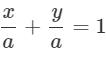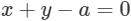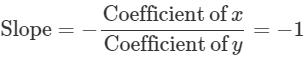QUESTION: 5

The points A and B have coordinates (3, 2) and (1, 4) respectively. So, the slope of any line perpendicular to AB is:

Solution:

If the lines are perpendicular to each other then their slopes are in the form m1.m2 = -1.(since product of slopes of two perpendicular lines is -1) Therefore , m = 1.

QUESTION: 6

Slope of a line is not defined, when q =

Solution:

Since tan θ is not defined when θ = 90°, therefore, the slope of a vertical line is not defined. i.e., slope of y-axis is m = tan 90° = ∞ i.e., not defined.

QUESTION: 7

If the slope of the line passing through the points (2, 5) and (x, 1) is 2, then x = ______.

Solution:

Slope = Change in y coordinates÷change in x coodinates
= (5-1)÷(2-x) =2
5-1 = 4-2x
0 = 2x
x=0

QUESTION: 8

If the slope of line m = tan 0°. Therefore, the line is _____ to the X-axis.

Solution:

Slope of x-axis is m = tan 0° = 0.
Since the inclination of every line parallel to x-axis is 0°, so its slope (m) = tan 0° = 0. Therefore, the slope of every horizontal line is 0.

QUESTION: 9

If A (-2, 1), B (2, 3) and C (-2, -4) are three points, find the angle between the straight lines AB and BC.

Solution:

Let the slope of the line AB and AC are m1 and m2  respectively.

Then,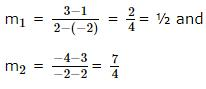Let θ be the angle between AB and BC. Then,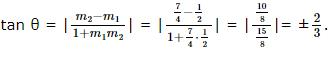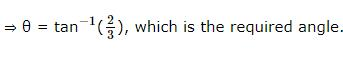QUESTION: 10

Let A(2, 12) and B(6,4) be two points. The slope of a line perpendicular to the line AB is:

Solution:

Slope of the points A and B is -2 and the lines are perpendicular then

m1×m2 = -1.

∴ the slope is 1/2Use Code STAYHOME200 and get INR 200 additional OFF Use Coupon Code

Track your progress, build streaks, highlight & save important lessons and more!

Similar ContentRelated tests